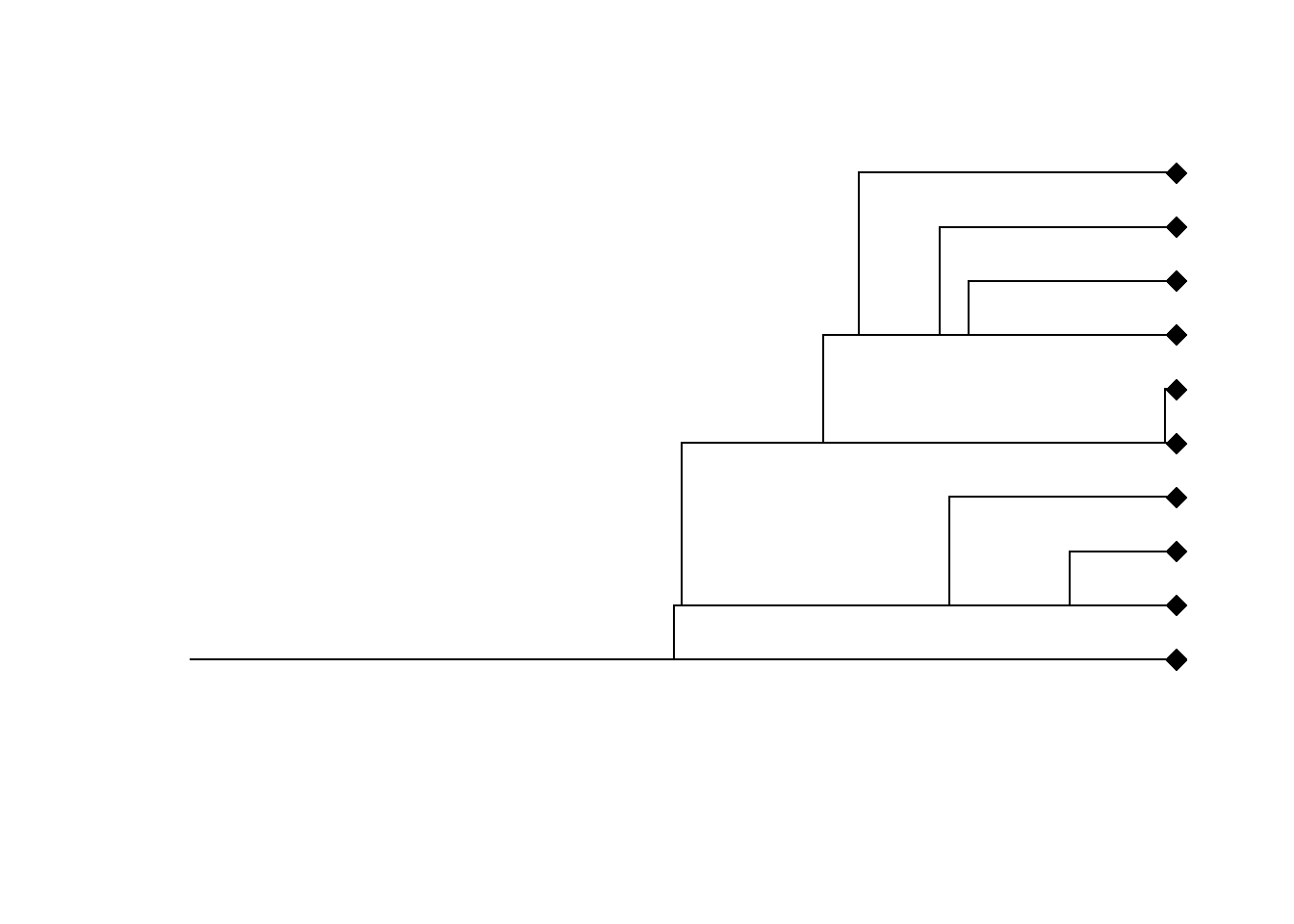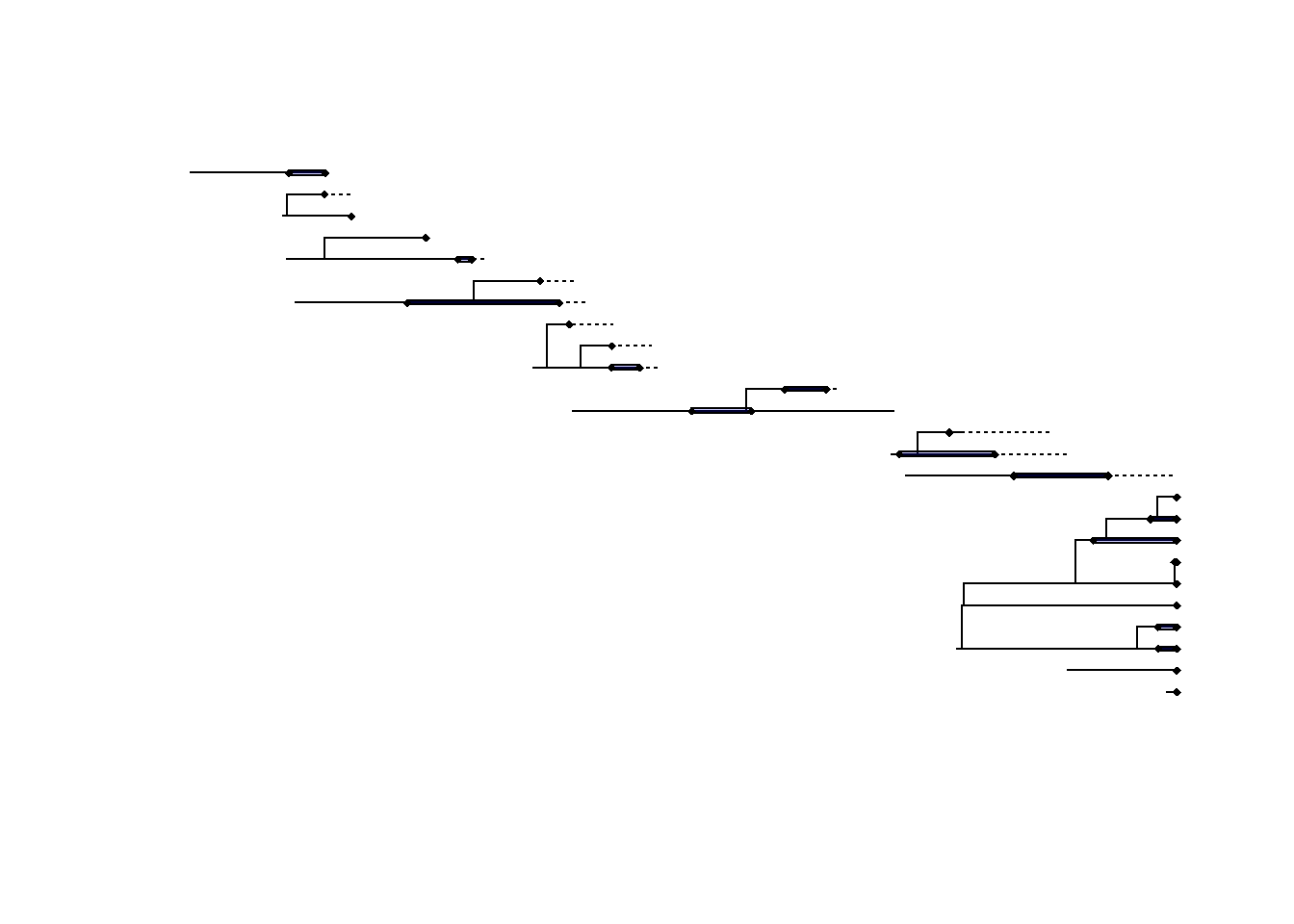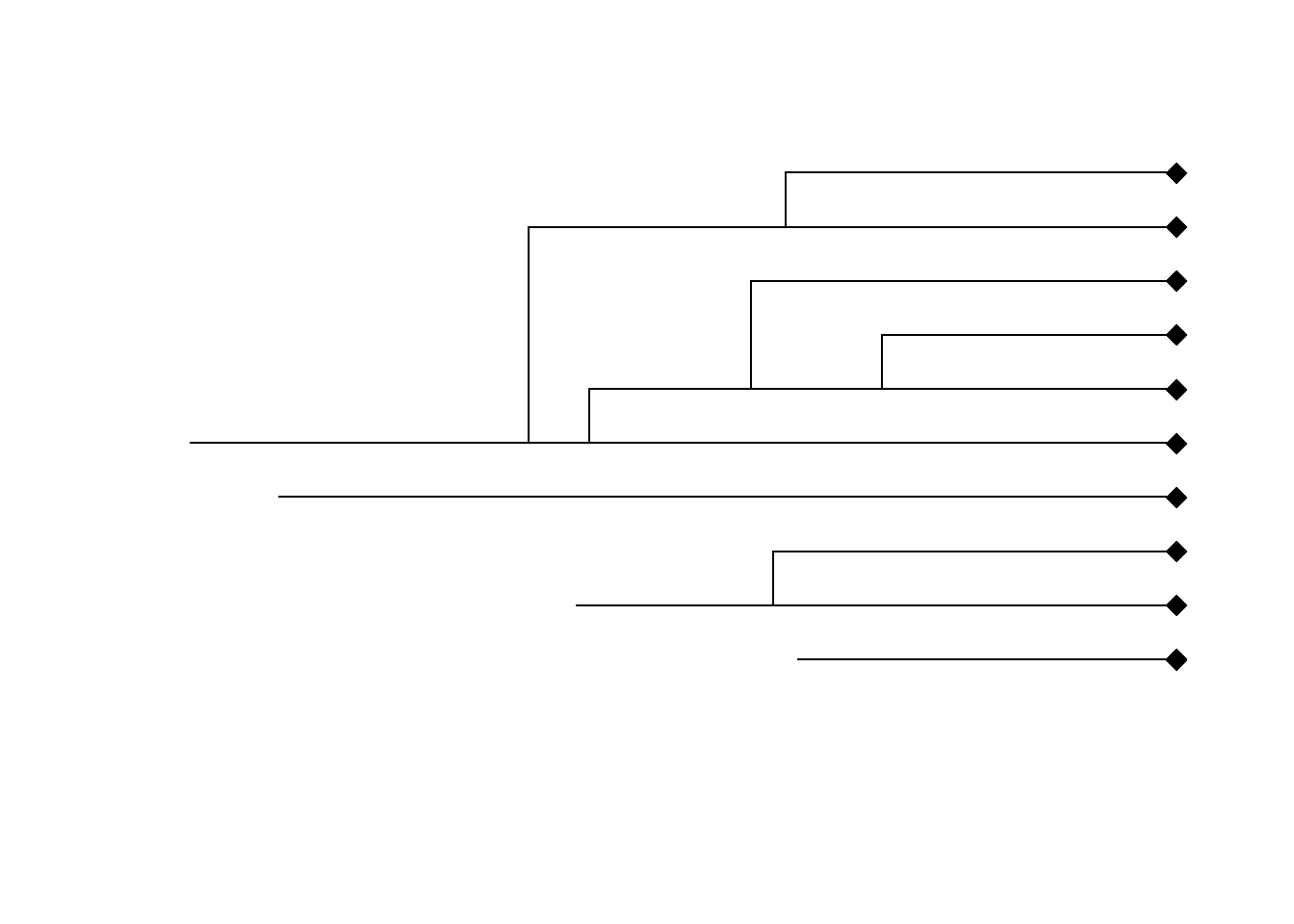## sim.fbd functions

FossilSim can be used to simulate trees directly from a fossilized-birth death process using the sim.fbd family of functions, whose interface is similar to the sim.bd functions in TreeSim. These functions return a list of SAtree objects, since the simulated trees may contain sampled ancestors represented as tips with zero-length branches.

## Simulating trees from a time-homogeneous fossilized-birth death process

As in TreeSim, trees can be simulated while conditioning either on the number of sampled extant taxa (using the sim.fbd.taxa function), or on the total age of the process (using the sim.fbd.age function). These functions require the fossil sampling rate parameter psi as an argument, but otherwise take the same arguments as the sim.bd.taxa and sim.bd.age functions in TreeSim.

trees <- sim.fbd.taxa(n=10, numbsim=10, lambda=3, mu=2, psi=2)

plot(trees[])Note that when simulating a complete tree (complete = TRUE), tips in the resulting SAtree object represent extinction events, whereas they represent fossil sampling events in the tree without unsampled lineages (complete = FALSE). The complete field of resulting the SAtree object is set to the value of the complete function argument.

trees <- sim.fbd.taxa(n=10, numbsim=10, lambda=3, mu=2, psi=2, complete=T)

print(trees[]\$complete)
##  TRUE
plot(trees[])## Simulating trees from an episodic fossilized-birth death process

Trees can also be simulated from a time-varying, piecewise constant fossilized birth-death process using the sim.fbd.rateshift.taxa function. Apart from a vector of interval specific psi parameters, this function also takes most of the same arguments as the corresponding sim.rateshift.taxa function in TreeSim. At the moment, this function assumes the sampling fraction of extant taxa (frac) is equal to 1.0.

n = 10
numbsim = 1
trees = sim.fbd.rateshift.taxa(n=10, numbsim=1, lambda=c(2,1), mu=c(0,0.3), psi=c(1,0.1), times=c(0.3))

plot(trees[])